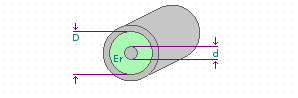# Le Leivre.com

## Coax Impedance Calculator

Calculate the impedance of a coaxial transmission line.D (mm) Er d (mm) Impedance

Notes:
*Optimal D/d ratio for breakdown voltage is 2.718.
*Optimal D/d ratio for power transfer is 1.65.
*Optimal D/d ratio for minimum attenuation is 3.59.
*Equation from Brian C. Wadell, “Transmission Line Design Handbook”